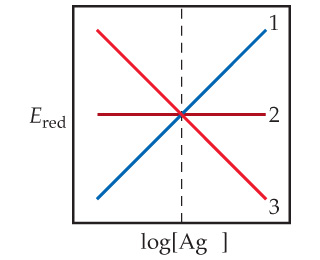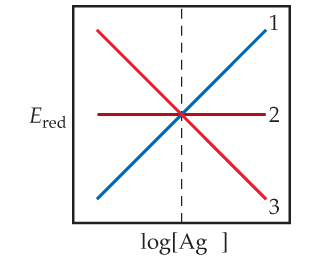# Problem: Consider the half reaction Ag+ ( aq ) + e-  →  Ag( s ).  Which of the lines in the following diagram indicates how the reduction potential varies with the concentration of Ag+?

###### FREE Expert Solution

We are asked which of the lines in the following diagram indicates how the reduction potential varies with the concentration of Ag+.The half-reaction for Ag+:

Ag+(aq) + e- → Ag(s)

91% (288 ratings)###### Problem Details

Consider the half reaction Ag+ ( aq ) + e-  →  Ag( s ).Which of the lines in the following diagram indicates how the reduction potential varies with the concentration of Ag+?